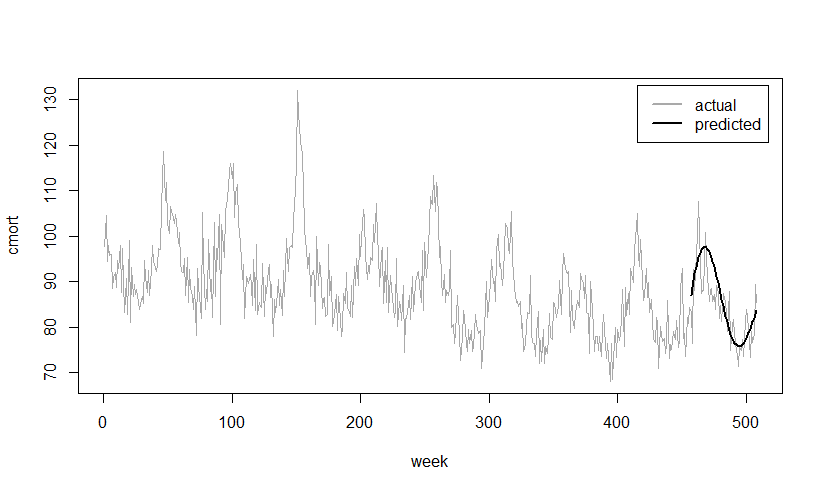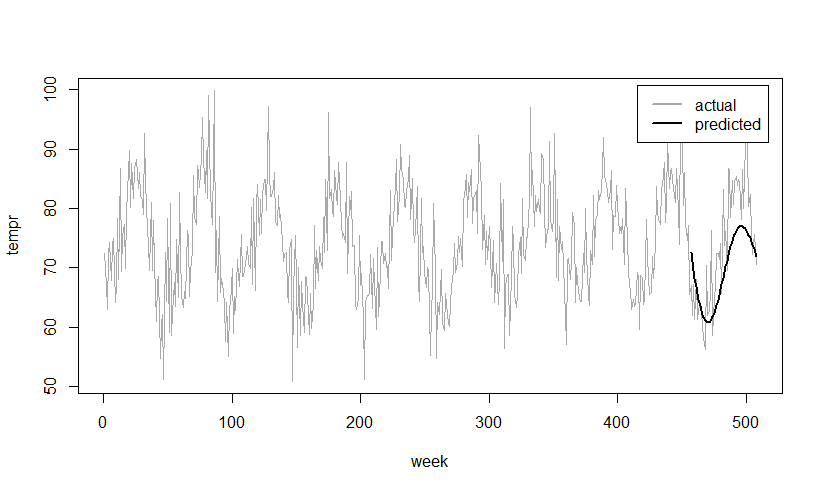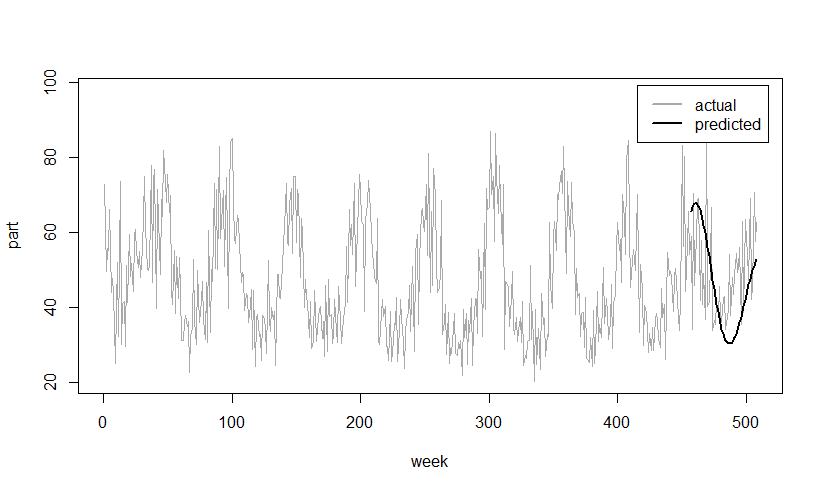# 7.00.02.01 - Using VARMAX with Aster R - Aster R

## Teradata Aster® R User GuideUpdate 3

prodname
Aster R
vrm_release
7.00.02.01
created_date
December 2017
category
Programming Reference
User Guide
featnum
B700-1033-700K
This section uses the dataset "lap", found in the R package "astsa". The dataset consists of 508 mean weekly observations of eleven variables including three measurements of human death rates (total mortality, cardiac-related mortality, and respiratory-related mortality) and eight atmospheric measurements (temperature, relative humidity, carbon monoxide, sulphur dioxide, nitrogen dioxide, hydrocarbons, ozone, and particulates).

This example uses the VARMAX function to predict three of the time series in the dataset: cardiac mortality ('cmort'), temperature ('tempr') and particulates ('part'). Users run the VARMAX function on a shortened version of the dataset – the first 456 points, and then plot the predicted values versus the actual values for the final fifty-two weeks for each of the three times series.

1. Convert the dataset from a time series (ts) object to an R data frame.
```library(astsa)
> class(lap)
 "mts" "ts"

> lap_df<-as.data.frame(lap)

> class(lap_df)
 "data.frame"```
2. Add 'seqid' and 'id' columns to prepare the data frame for use with the VARMAX function.
```seqid <- rep(1, 508)
id <- seq(1:508)
lap_df_indexed <- cbind(seqid, id, lap_df)```
3. Create a table in the Aster Database to hold the data.
```ta.dropTable(“lap_data”)

ta.create(lap_df_indexed,
table="lap_data",
schemaName="public",
tableType="dimension",
row.names=TRUE,
colTypes=NULL
)```
4. Create the virtual data frames, using the first 456 points of the dataset.
```tadf_lap <- ta.data.frame('lap_data')
```
5. Call the VARMAX function.
```result <- aa.varmax(data=tadf_lap_short,
data.partition.col='seqid',
data.order.col='id',
response.columns=c('cmort','tempr','part'),
partition.columns='id',
orders='1,0,1',
)
```
6. The result is an object of class "aa.varmax", similar to an R list object. Uses can access the results using the result[] command. The results include the VARMAX coefficients, goodness of fit measurements, and whether the function converged, in addition to the fifty-two predicted values for the three response columns.
7. Convert the result to an R data frame.
`results_df <- as.data.frame(result[])`
8. Retrieve the step ahead predictions.
```cmort_pred <- results_df[14:65, 'predict_cmort']
tempr_pred <- results_df[14:65, 'predict_tempr']
part_pred <- results_df[14:65, 'predict_part']```
9. Create a data frame consisting of the first 456 values of 'cmort', 'tempr', and 'part' with the actual and predicted values for the final fifty-two values.
```df_short<-lap[1:456,c("cmort","tempr","part")]
df_na<-rep(NA, 456)

df_pred<-cbind(cmort_pred, tempr_pred, part_pred)
df_actual<-lap[457:508,c("cmort","tempr","part")]

weeks_1to456 <- cbind(df_short, df_na, df_na, df_na)
weeks_457to508 <-cbind(df_actual, df_pred)

df_complete<-rbind(weeks_1to456, weeks_457to508)```
10. Convert the data frame to class "time series" to use the time series plotting functions.
`ts_complete <- ts(df_complete)`
11. Plot the results.
1. Plot the 'cmort' (cardiac mortality) data to compare the predicted values to the actual values.
```ts.plot(ts_complete[,c(1,4)], gpars=list(col=c("darkgray","black"),lwd=c(0.5,2.5), ylab="cmort", xlab="week"))
legend("topright", lty = 1, lwd=2.5,col=c("darkgray","black"),legend=c("actual","predicted"), inset = .02)
```2. Plot the 'tempr' (temperature) data to compare the predicted values to the actual values.
```ts.plot(ts_complete[,c(2,5)], gpars=list(col=c("darkgray","black"),lwd=c(0.5,2.5), ylab="tempr", xlab="week"))
legend("topright", lty = 1, lwd=2.5,col=c("darkgray","black"),legend=c("actual","predicted"), inset = .02)
```3. Plot the 'part' (particulates) data to compare the predicted values to the actual values.
```ts.plot(ts_complete[,c(3,6)], gpars=list(col=c("darkgray","black"),lwd=c(0.5,2.5), ylab="part", xlab="week"))
legend("topright", lty = 1, lwd=2.5,col=c("darkgray","black"),legend=c("actual","predicted"), inset = .02)
```